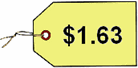Skill: 2F Money: Making Change Normally, a student would calculate the amount of change given by writing a simple subtraction problem on paper subtracting the "cost amount" from the "pays with" amount. However, in the "real-world" - paper and pencil calculations are not practical when making a puchase at the store. Therefore, it is important to learn how to "count-up" to find the difference in two numbers, as in determining the amount of change to receive.  Your student should determine this answer by drawing the actual dollars and coins on paper and should be "counting-up" to get the answer.  If he/she must write a subtraction problem to get the answer then this skill needs to be practiced.  THEY MUST KNOW HOW TO COUNT UP TO DETERMINE THE CORRECT CHANGE! Martha buys a package of paper for \$1.63. She pays for it with a \$5 bill.  How much change should she get? Your answer should use the fewest bills and coins possible. CostsPays with \$5.00 Start with \$1.63. Then count up. Draw the coins and bills as you count up to \$5.00 What is the total value of Martha's change? ________